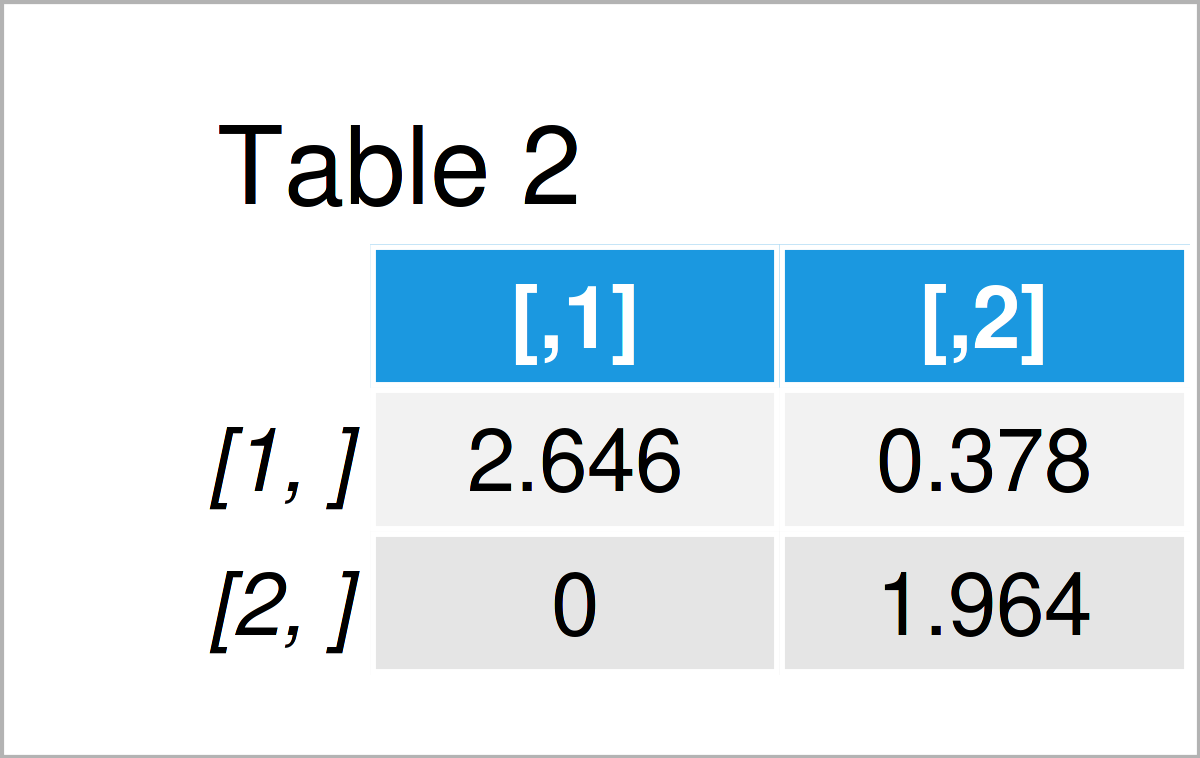# Choleski Decomposition in R (Example) | Factorization with chol() Function

In this R tutorial you’ll learn how to conduct a Choleski decomposition (or factorization) using the chol function.

You’re here for the answer, so let’s get straight to the example.

## Constructing Example Data

First, we need to create some data that we can use in the following examples:

```my_mat <- matrix(c(7, 1, 1, 4), nrow = 2)    # Create example matrix
my_mat                                       # Print example matrix```Have a look at the previous table. It shows that our example data matrix has two rows and two numerical variables.

## Example: Perform Choleski Decomposition Using chol() Function

The following syntax illustrates how to apply the chol function to conduct a Choleski decomposition in R.

Consider the following R code:

```my_mat_chol <- chol(my_mat)                  # Apply chol function
my_mat_chol                                  # Print output matrix```As shown in Table 2, we have created a new matrix object containing the Choleski factorization of our input matrix.

## Video & Further Resources

In case you need further explanations on the R codes of the present page, I recommend watching the following video on my YouTube channel. In the video, I’m explaining the contents of this post:

Furthermore, you may read the related articles on my website. I have published several articles already.

To summarize: You have learned in this article how to apply the chol function in R programming. Don’t hesitate to let me know in the comments, in case you have further questions.

Subscribe to the Statistics Globe Newsletter